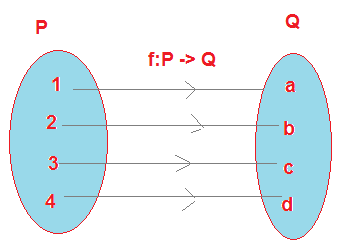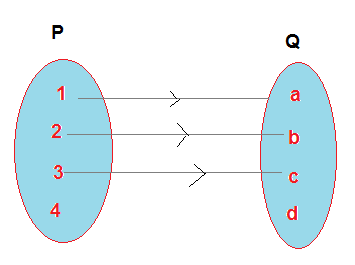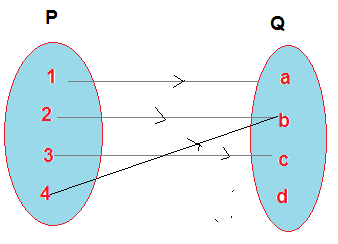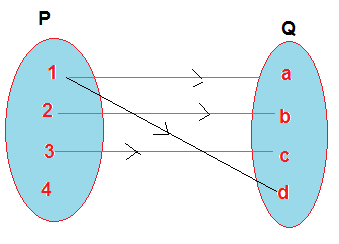# Functions in Maths

## 3. What is Function

• A function is a "well-behaved" relation
• A function $f$ is a relation from a non-empty set $A$ to a non-empty set $B$ such that the domain of $f$ is $A$ and no two distinct ordered pairs in $f$ have the same first element.
• For a relation to be a function, there must be only and exactly one $y$ that corresponds to a given $x$
• If $f$ is a function from $A$ to $B$ and $\left( {a,b} \right) \in f$, then $f\left( a \right) = b$, where $b$ is called the image of $a$ under $f$ and $a$ is called the pre-image of $b$ under$f$.
• A function is also termed as a map or a mapping
• A function from A to B is denoted as f: A -> B
• we often denote function as y=f(x). Here y is the function of x. x is called the independent variable and y is called the dependent variable.

### Function Notation

Function are generally denoted by
a. f: A -> B . This tells that ordered pair is formed by elements of A and elements . This is called arrow notation
b. Another popular way to notate function is f(x). Here f(x) is called function(f) of x. We can express like the formula
$f(x) = x^2 +2$
$f(x) = x + 6$
We can also denote this as y=f(x)
Here y is the function of x. x is called the independent variable and y is called the dependent variable
Example 1:
Which of the following relations are functions? Give reasons. If it is a function, determine its domain and range.
1. $\left\{ {\left( {3,1} \right),\left( {5,1} \right),\left( {7,1} \right),\left( {11,1} \right),\left( {14,1} \right),\left( {17,1} \right)} \right\}$
2. ${\left\{ {\left( {2,1} \right),\left( {4,2} \right),\left( {6,3} \right),\left( {6,4} \right),\left( {10,5} \right),\left( {12,6} \right),\left( {14,7} \right)} \right\}}$
3. ${\left\{ {\left( {1,3} \right),\left( {1,5} \right),\left( {2,5} \right)} \right\}}$
1. $\left\{ {\left( {3,1} \right),\left( {5,1} \right),\left( {7,1} \right),\left( {11,1} \right),\left( {14,1} \right),\left( {17,1} \right)} \right\}$
Since 3, 5, 8, 11, 14, and 17 are the elements of the domain of the given relation having their unique images, this relation is a function.
2. ${\left\{ {\left( {2,1} \right),\left( {4,2} \right),\left( {6,3} \right),\left( {6,4} \right),\left( {10,5} \right),\left( {12,6} \right),\left( {14,7} \right)} \right\}}$
Since the same first element i.e 6 corresponds to two different images 3 and 4, this relation is not a function
3. ${\left\{ {\left( {1,3} \right),\left( {1,5} \right),\left( {2,5} \right)} \right\}}$
Since the same first element i.e., 1 corresponds to two different images i.e., 3 and 5, this relation is not a function.
Example 2:

Let P ={1,2,3,4} and Q={11,12,13,14}. Relation are defined from P to Q as R:P-> Q
a. R ={(1,11),(2,12),(3,13),(4,14)}
b. R ={(1,11),(2,12),(3,13)}
c. R ={(1,11),(2,11),(3,11), (4,11)}
d. R ={(1,11),(1,11),(3,11), (4,11),(4,14)}
Which of the following relations are functions? Give reasons.
a. A function as each element in P has corresponding image in Q
b. Not a function because there is no element in Q which corresponds to element 4 in P
c. A function
d. Not a function as element has multiple images in Q

## Pictorial representation of Functions

Just like relations, functions are also represented pictorially using arrow diagram
Let P ={1,2,3,4} and Q={a,b,c,d}. function is defined from P to Q as f:P-> Q
f={(1,a),(2,b),(3,c),(4,d)}
Arrow diagram for this function will beWe can identify which relations are function from arrow diagram also. Lets check out some exampleThe above is not a functionThe above is a functionThe above is not a function

## Real valued function and Real Functions

A function which has either R or one of its subsets as its range is called a real valued function. Further, if its domain is also either R or a subset of R, it is called a real function.

Example
1. Function f: R -> R defined by f(x) =$2x+1$ for each $x \in R$ is a real function
2. Function f:N -> N defined by f (x) = $3x + 1$ for each $x \in N$ is a real function

## Examples of Functions in Maths

1. Let A ={1,2,3,4}. A relation R is defined from X to N
$R={(x,y),y=x^3 + 2, x \in A ,y \in N }$
Determine if this relation is a function?
Solution
Here $R={(x,y),y=x^3 + 2, x \in A ,y \in N }$
Now x takes the values as 1,2,3,4
Therefore for x= 1 y = 1 +2=3
for x=2 , y=8+2 =10
for x=3,y=27+2=29
for x=4, y=64+2=66
So relation can be written as
R={(1,3),(2,10),(3,29),(4,66)}
Here We can see that each element of A is the first element of some ordered pair in the relation and no element in A has multiple images in R
Hence it is a function

2.Let N be the set of natural numbers and the relation R be defined on N
$R = {(x, y) : y = 2x, x, y \in N}$
Is this relation a function?
Solution
Here The domain of R is the set of natural numbers N. The co-domain is also N.
The range is the set of even natural numbers.
Since every natural number n has one and only one image, this relation is a function.

### Quiz Time

Question 1.
which is of the below relation is not a function.
A. R = {(2,2),(2,4),(3,3), (4,4)}
B. R = {(2,1),(3,1), (4,2)}
C. R = {(1,2),(2,3),(3,4), (4,5), (5,6), (6,7)}
D. None of the above
Question 2.
Let A = {1, 2, 3,4} and B = {5, 7}. Then possible number of relation from A to B ?
A. 64
B. 256
C. 16
D. 32
Question 3.
if function f : X -> R, $f (x) = x^3$, where X = {-1, 0, 3, 9, 7}, the function expressed in ordered pair will be
A. {(-1,-1),(1,1),(3,27), (7,343),(9,729)}
B. {(-1,-1),(0,0),(3,27)}
C. {(-1,1),(0,0),(3,9), (7,343),(9,729)}
D. {(-1,-1),(0,0),(3,27), (7,343),(9,729)}
Question 4.
Let f(x) =x2 ,find the value of $\frac {f(2.1) -f(2)}{2.1 -2}$
A. 4
B. .41
C. 4.1
D. .14
Question 5.
Find the range of the function defined as $f(x)=\sqrt {9-x^2}$
A. [-3,3]
B. [0,3]
C.(0,3)
D.[-3,0)
Question 6.
Find the domain of the function $f(x) =\frac {x^2+1}{x^2-5x+4}$
A. R
B. R - {4}
C. R - {1}
D. R -{1,4}